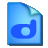Quick Calculate

1.3

Quick Calculate is a wonderful free game which helps anyone to develop an interest in solving mathematical problems. It has a very simple and interesting user interface.

5
Rated by
2 users
Freeware
OS:
Windows 7
Date:
Category rank:
573
All software rank:
25 576

More about Quick Calculate

You can download the setup package 259.12 KB of Quick Calculate v1.2 from this database. Novel Games Limited provides this program for free. You can find it in Education category, namely Science. The mostly used program version running on Windows 7 is 1.1. The statistics show that Quick Calculate.exe and quickcalculate.exe are among the most popular setup files for the software. The direct download link has been checked by our antiviruses and has been found safe.Download
Quick Calculate 1.3

Comments on Quick Calculate

Thank you for rating the program!
Please add a comment explaining the reasoning behind your vote.

Useful softwareSmart Math Calculator
Define variables and solve mathematical equations and functions.MathType
Edit mathematical equations for Word files, web pages, and LaTeX documents.